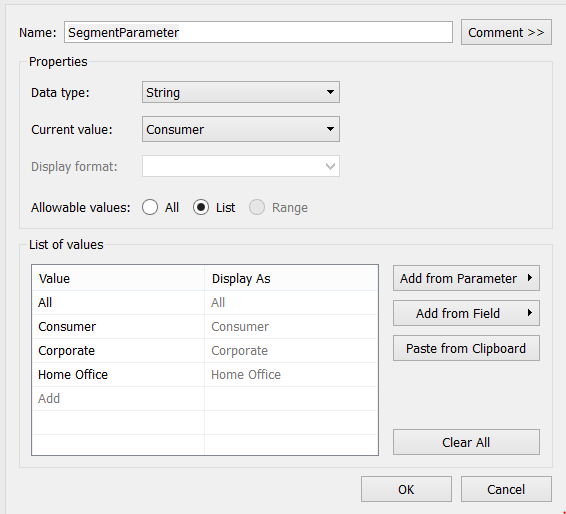# Simple way to add All to a parameter in Tableau

Back in 2012, I wrote a post describing an overly complex way to add ‘All’ to a parameter in Tableau. It is surprisingly simple to add All to a parameter if using the parameter to select a value from a dimension.

First create a patameter and add the values from the field and the value All.

Next create a calculated field to use the parameter. The generic formula to use ‘All’ in a Tableau parameter is:

[Parameter] = ‘All’ OR [Parameter] = [DimensionName]

The following is a very simple example based on the Superstore data. In this example the Segment is selected from a parameter, called SegmentParameter.

The parameter is built from the Segment field (Add from Field) and All was also added.A calculated field is needed to act as a filter, making use of the parameter. The formula used within the calculated field is:

[SegmentParameter] = ‘All’ OR [SegmentParameter] = [Segment]

Place this calculated field on this filters shelf and set to ‘True’. Simple as that, All is now in the Tableau parameter.

##### How does this work?

First look at the case where a segment is selected. When a segment is chosen in the filter True is only returned for that segment, for example if Consumer is selected the calculated field returns True where

(in parameter) Consumer = Consumer (in dimension)

False is returned for all other segments – i.e.

(in parameter) Consumer = Corporate (in dimension)

is False as consumer clearly does not equal corporate.

Therefore only the Consumer value is displayed.

When All is selected the calculated field returns the value True as All = All. This actually does nothing to prevent any segments from being displayed, therefore all Segments are displayed. The OR in the calculated field is key here as it means the second part of the calculated field is irrelevant as True has already been returned.

2018-05-07T07:35:30+00:00 October 3rd, 2016|Tableau|

This site uses Akismet to reduce spam. Learn how your comment data is processed.